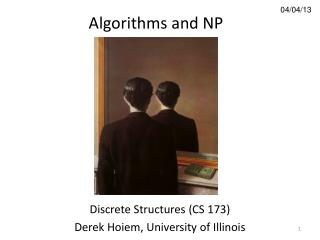DownloadDownload Presentation04/04/13

# 04/04/13

Télécharger la présentation## 04/04/13

- - - - - - - - - - - - - - - - - - - - - - - - - - - E N D - - - - - - - - - - - - - - - - - - - - - - - - - - -
##### Presentation Transcript

1. Algorithms and NP 04/04/13 Discrete Structures (CS 173) Derek Hoiem, University of Illinois

2. Administrative • Mini-homework released yesterday • Long-form homework • Problems released on website today • Moodle boxes available on Friday • Note: mini-hw is long, and long-form homework is short • Tests will be released next week’s discussion • Do not discuss with those who haven’t taken it yet

3. This class • Review of algorithms and big-O • Computing factorial series • Multiplying large numbers • The master theorem • Algorithmic complexity • P vs. NP

4. Example: factorialSeries.m Lesson 1: Be careful of implementation details that hide computational complexity Lesson 2: Knowing complexity of algorithm can help find major implementation flaws See code

5. Master theorem

6. Master theorem Leaf term dominates (hyper expansion) If with , then Each level costs the same (balanced expansion) If with , then Top node dominates (slow expansion) If with , then

7. Master theorem If with , then Example: Example algorithm: multiplying large numbers

8. Master theorem If with , then Example: Example algorithm: sorting

9. Master theorem If with , then Example:

10. Example: multiplying large numbers Multiplying small numbers in binary Multiplying large numbers Complexity: Complexity:

11. Example: multiplying large numbers Multiplying large numbers Trick by AnatoliiKaratsuba Complexity:

12. Algorithm complexity constant, sublinear, linear, linearithmic, quadratic, cubic, exponential, factorial time problem size http://en.wikipedia.org/wiki/Computational_complexity_of_mathematical_operations

13. Average-case vs. worst-case complexity • Sometimes worst case is unlikely or avoidable • E.g., quicksort • Average-case complexity describes behavior for a typical case

14. P vs. NP A problem is class P if a polynomial-time solution exists A problem is class NP (non-deterministic polynomial time) if a solution can be checked in polynomial time, but no known algorithm can generate the solution in polynomial time

15. Examples Boolean satisfiability: Determine if any assignment of boolean variables can satisfy a set of logical expressions E.g., is 3-SAT problem How fast can you find a solution? How fast can you check a solution? NP: finding solution takes exponential time, but checking solution is polynomial

16. Examples Sorting a set of integers How fast can you find a solution? How fast can you check a solution? P: sorting takes linearithmic time, and checking takes linear time

17. Examples Finding the chromatic number • Determining if the graph is -colorable • Determining if the graph is not -colorable • is in NP, can be checked quickly • is in NP-hard (not necessarily in NP)

18. P = NP? • If a problem can be checked efficiently (class P), then can we solve it efficiently? • Yes: • No: • Introduced by Stephen Cook in 1971 • Cook, one of the founders of computational complexity, was denied tenure by Berkeley in 1969 • Notion of NP-complete introduced in the same paper • Proof is worth \$1,000,000 (Millenium Prize Problem)

19. NP-complete and NP-hard Any NP problem can be reduced in polynomial time to an NP-completeproblem - Example: satisfiability If any NP-completeproblem can be solved in polynomial time, then all NPproblems can be solved in polynomial time A problem is NP-hard if an NP-complete problem can be reduced to it If then no NP-complete algorithm can be solved in polynomial time

20. Examples Traveling salesman problem: determine an order of cities to visit that minimizes total travel time NP-hard: finding solution takes exponential time, checking solution is NP-complete

21. Things to remember • Be able to analyze code for computational cost • Tools: finding loops and recursive calls, using recursion trees • Sometimes need to know inner-workings of a library to determine (e.g., factorialSeries) • Be able to convert to big-O or big-Theta and be familiar with basic complexity terms • E.g., linear, nlogn, polynomial, exponential • Problems in NP can be checked in polynomial time but probably not solved in polynomial time • P=NP is open problem, most think not